This function calls mkinfit on all combinations of models and datasets specified in its first two arguments.

## Usage

mmkin(
models = c("SFO", "FOMC", "DFOP"),
datasets,
cores = if (Sys.info()["sysname"] == "Windows") 1 else parallel::detectCores(),
cluster = NULL,
...
)

# S3 method for mmkin
print(x, ...)

## Arguments

models

Either a character vector of shorthand names like c("SFO", "FOMC", "DFOP", "HS", "SFORB"), or an optionally named list of mkinmod objects.

datasets

An optionally named list of datasets suitable as observed data for mkinfit.

cores

The number of cores to be used for multicore processing. This is only used when the cluster argument is NULL. On Windows machines, cores > 1 is not supported, you need to use the cluster argument to use multiple logical processors. Per default, all cores detected by parallel::detectCores() are used, except on Windows where the default is 1.

cluster

A cluster as returned by makeCluster to be used for parallel execution.

...

Not used.

x

An mmkin object.

## Value

A two-dimensional array of mkinfit

objects and/or try-errors that can be indexed using the model names for the first index (row index) and the dataset names for the second index (column index).

[.mmkin for subsetting, plot.mmkin for plotting.

Johannes Ranke

## Examples


# \dontrun{
m_synth_SFO_lin <- mkinmod(parent = mkinsub("SFO", "M1"),
M1 = mkinsub("SFO", "M2"),
M2 = mkinsub("SFO"), use_of_ff = "max")
#> Temporary DLL for differentials generated and loaded

m_synth_FOMC_lin <- mkinmod(parent = mkinsub("FOMC", "M1"),
M1 = mkinsub("SFO", "M2"),
M2 = mkinsub("SFO"), use_of_ff = "max")
#> Temporary DLL for differentials generated and loaded

models <- list(SFO_lin = m_synth_SFO_lin, FOMC_lin = m_synth_FOMC_lin)
datasets <- lapply(synthetic_data_for_UBA_2014[1:3], function(x) x$data) names(datasets) <- paste("Dataset", 1:3) time_default <- system.time(fits.0 <- mmkin(models, datasets, quiet = TRUE)) time_1 <- system.time(fits.4 <- mmkin(models, datasets, cores = 1, quiet = TRUE)) time_default #> user system elapsed #> 1.653 0.553 0.654 time_1 #> user system elapsed #> 2.013 0.008 2.021 endpoints(fits.0[["SFO_lin", 2]]) #>$ff
#>   parent_M1 parent_sink       M1_M2     M1_sink
#>   0.7340481   0.2659519   0.7505690   0.2494310
#>
#> \$distimes
#>              DT50       DT90
#> parent  0.8777689   2.915885
#> M1      2.3257403   7.725942
#> M2     33.7201060 112.015767
#>

# plot.mkinfit handles rows or columns of mmkin result objects
plot(fits.0[1, ])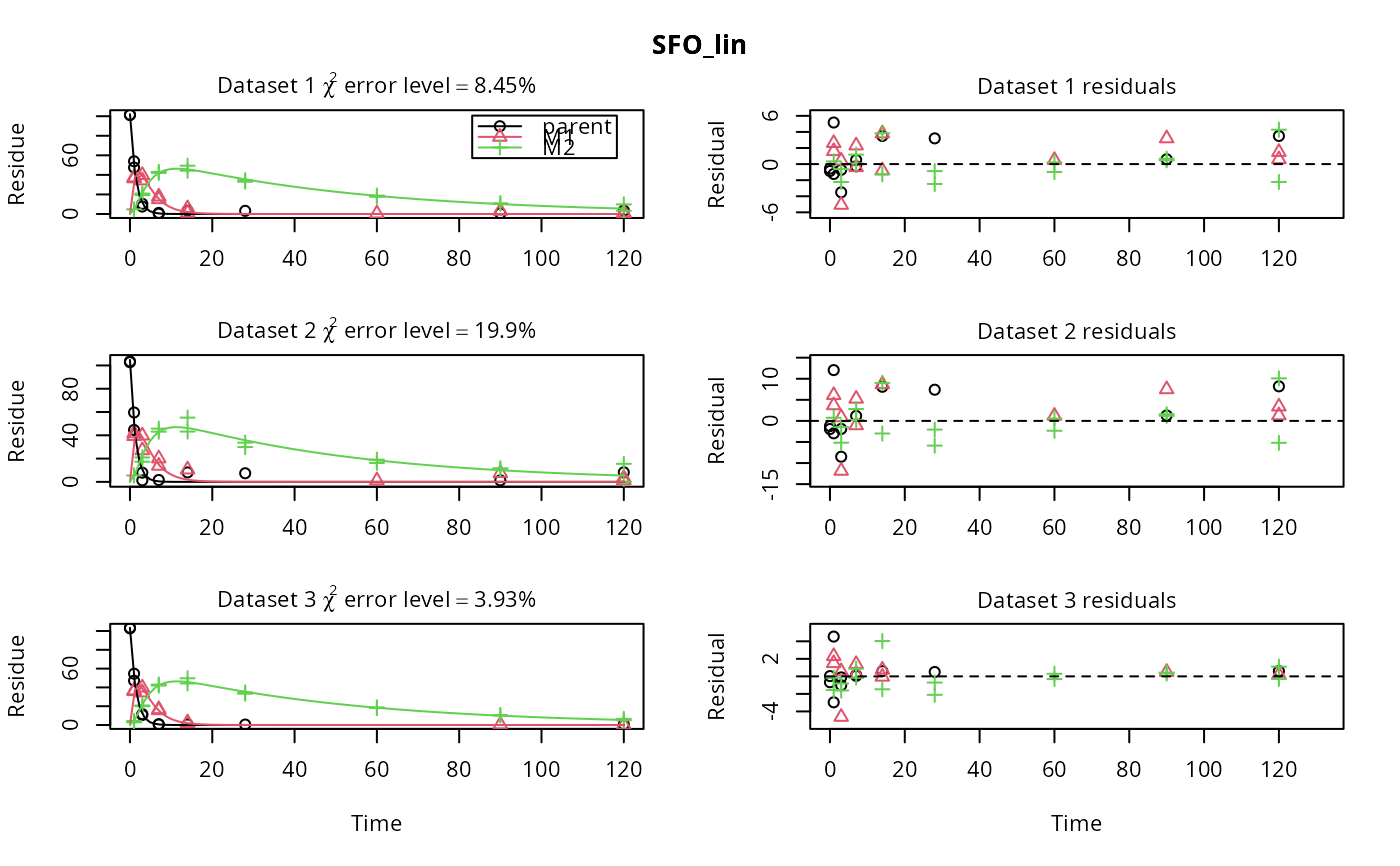plot(fits.0[1, ], obs_var = c("M1", "M2"))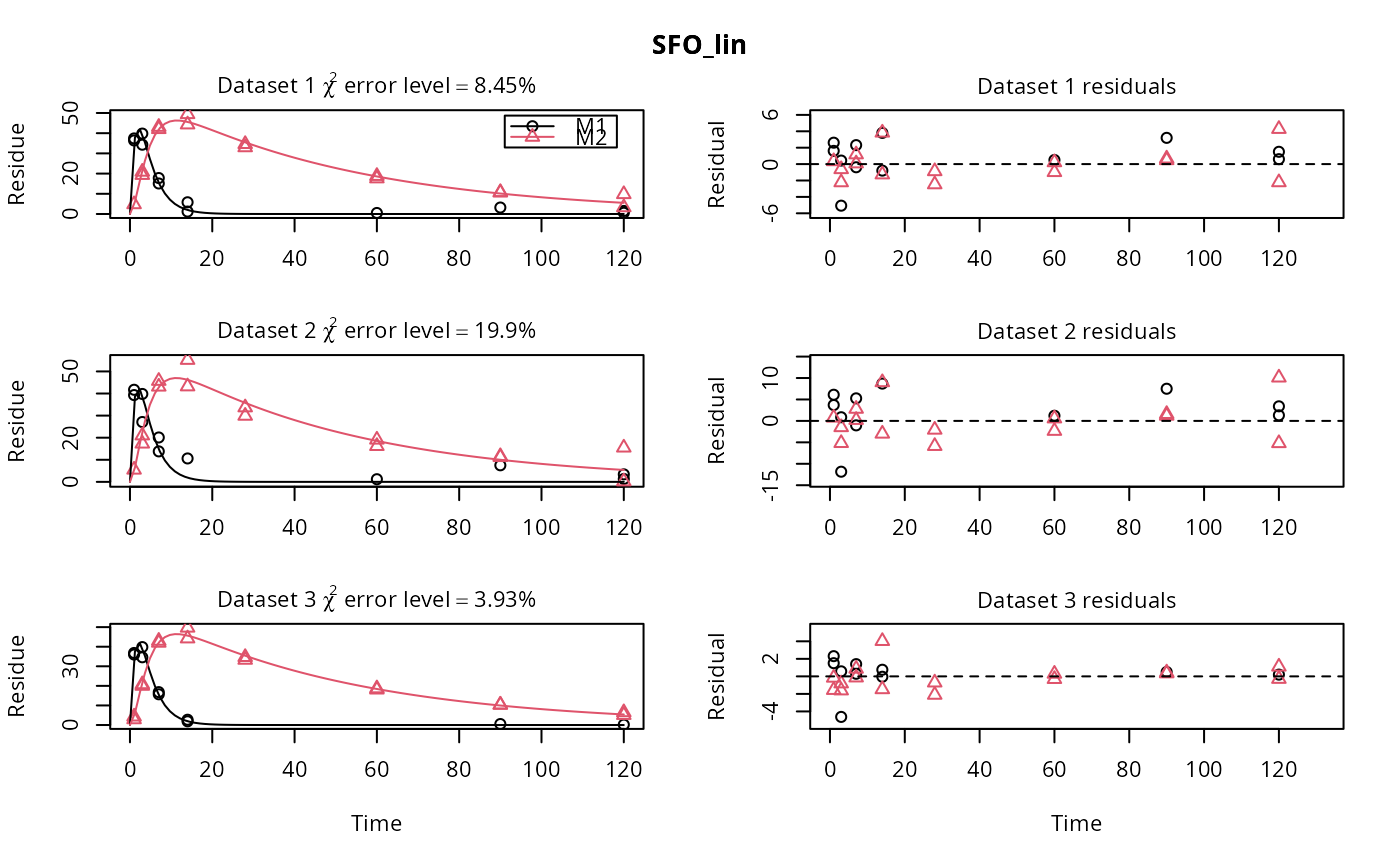plot(fits.0[, 1])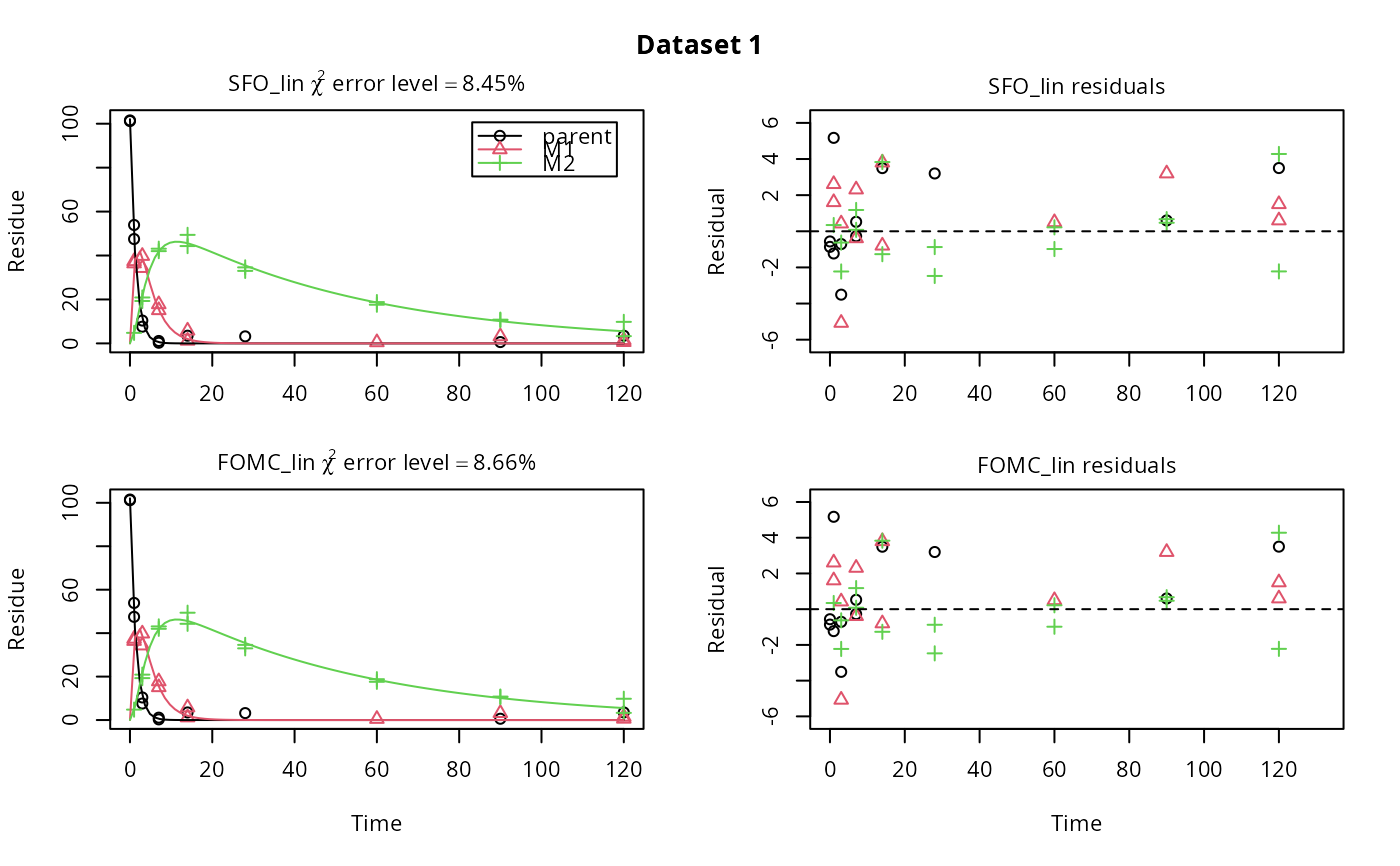# Use double brackets to extract a single mkinfit object, which will be plotted
# by plot.mkinfit and can be plotted using plot_sep
plot(fits.0[[1, 1]], sep_obs = TRUE, show_residuals = TRUE, show_errmin = TRUE)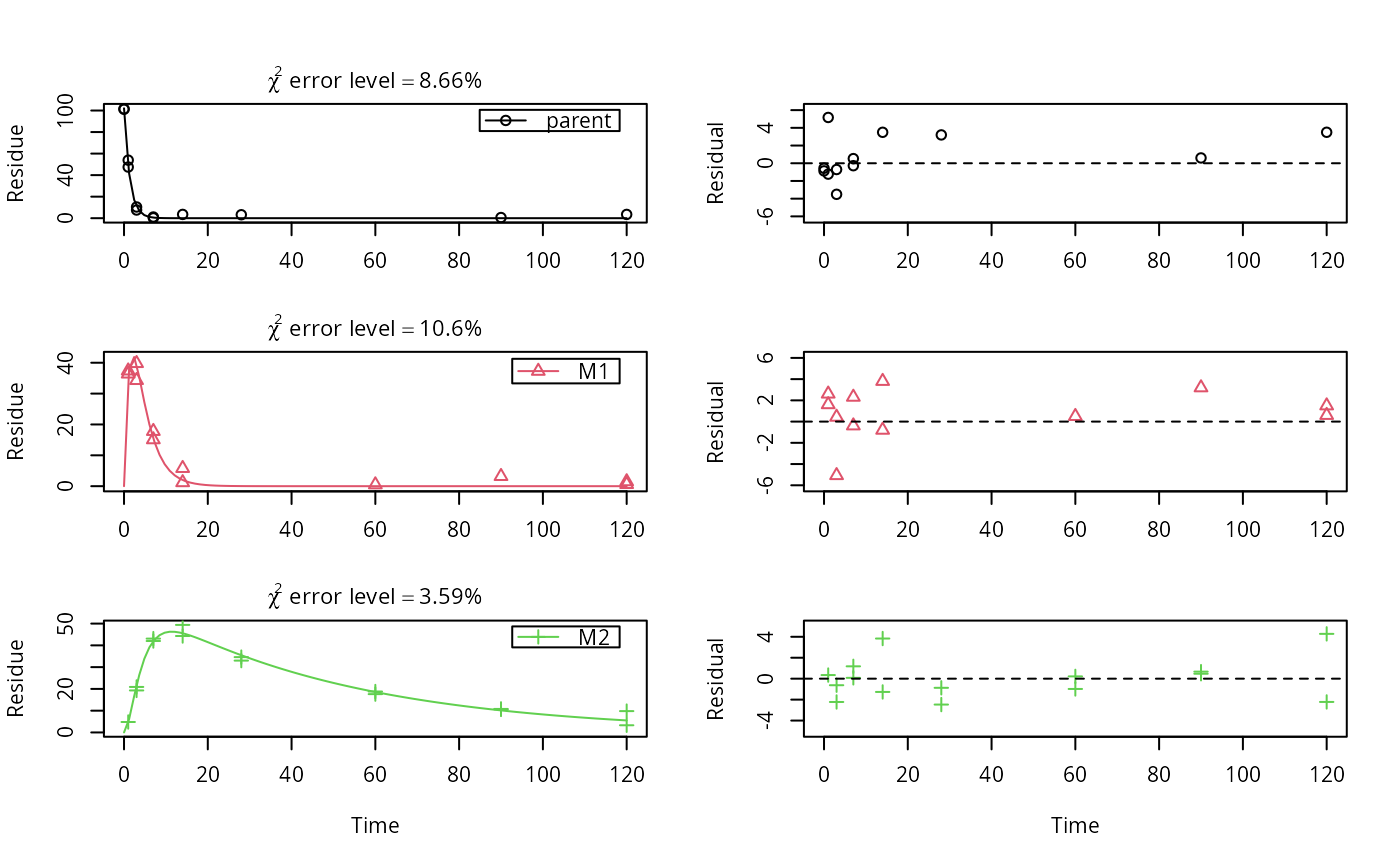plot_sep(fits.0[[1, 1]])
# Plotting with mmkin (single brackets, extracting an mmkin object) does not
# allow to plot the observed variables separately
plot(fits.0[1, 1])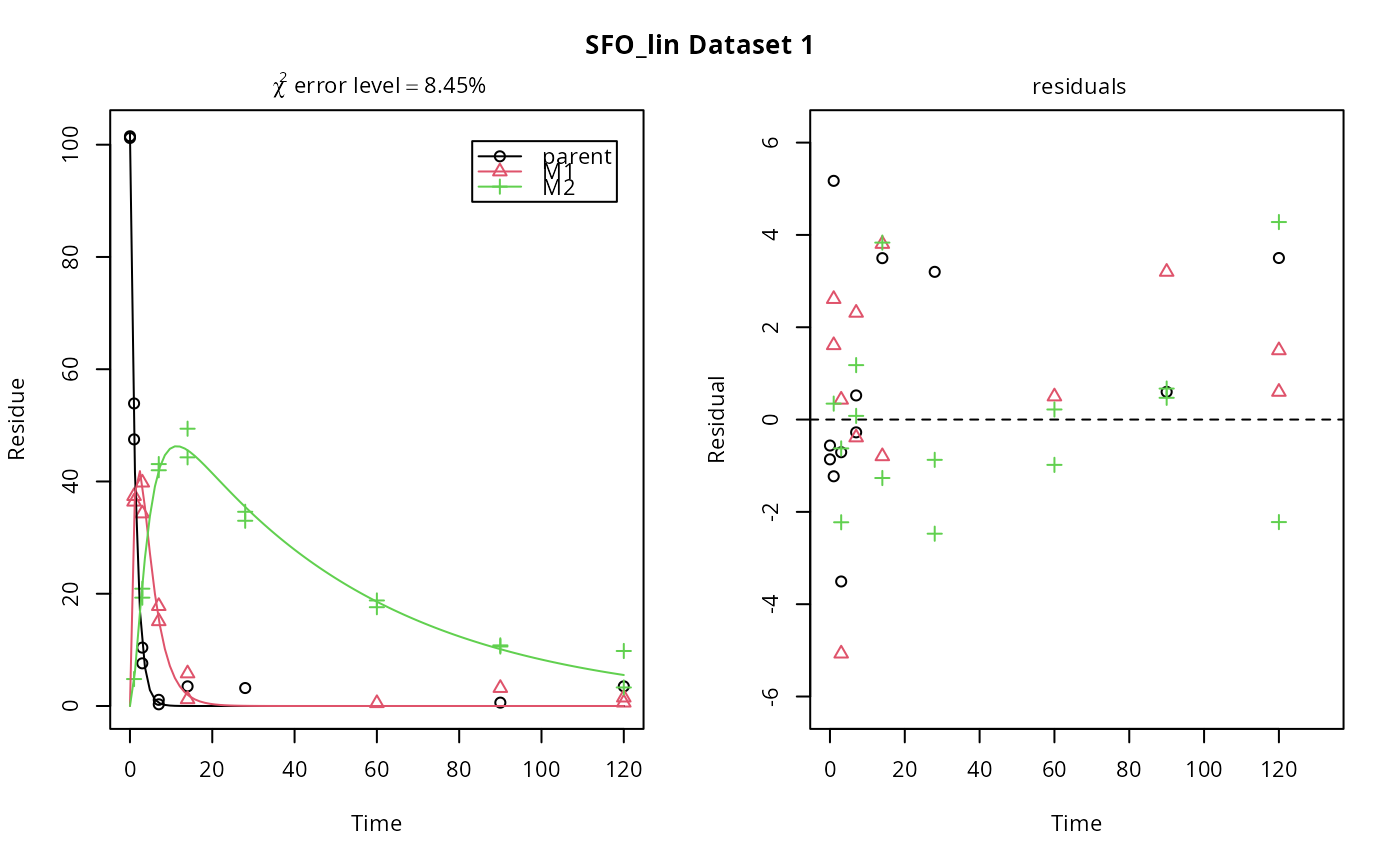# On Windows, we can use multiple cores by making a cluster first
cl <- parallel::makePSOCKcluster(12)
f <- mmkin(c("SFO", "FOMC", "DFOP"),
list(A = FOCUS_2006_A, B = FOCUS_2006_B, C = FOCUS_2006_C, D = FOCUS_2006_D),
cluster = cl, quiet = TRUE)
print(f)
#> <mmkin> object
#> Status of individual fits:
#>
#>       dataset
#> model  A  B  C  D
#>   SFO  OK OK OK OK
#>   FOMC C  OK OK OK
#>   DFOP OK OK OK OK
#>
#> C: Optimisation did not converge:
#> false convergence (8)
#> OK: No warnings
# We get false convergence for the FOMC fit to FOCUS_2006_A because this
# dataset is really SFO, and the FOMC fit is overparameterised
parallel::stopCluster(cl)
# }